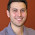## Tuesday, April 5, 2011

### How to Unroll a For-Loop in C++

In this article we will show you how to manually unroll a loop in C++. Loop unrolling has performance advantages due to the reduced overhead of checking and advancing the loop counter at each iteration. Also, when using vectorized mathematical operations such as `SSE` instructions, it is possible to perform some iterations of the same loop in parallel. Let's first focus on the general concept of loop unrolling with a simple example:
```int* x = /* some pointer to data */;
const int N = /* number of elements in x */;
for(int i = 0; i < N; i++)
{
foo(x[i]);
}
```
You often see examples where people unroll loops in an unsafe way:
```for(int i = 0; i < N; i+=2)
{
foo(x[i + 0]);
foo(x[i + 1]);
}
```
Executing such an unrolled loop will be fine for any `N` that is a multiple of 2. But what happens if `N` is odd? You will access some data that you do not own. For example, if `N=3`, you will enter the body of the loop twice (with `i=0` and `i=2`). At the second iteration you will access the element `x` of a 3-element array. In the worst case this leads to an access violation or at least you access some uninitialized data.

Therefore, if you can not assume that `N` is a multiple of you step size, you need to take care of the general case. The best way for doing that is to have an unrolled version of your loop for all elements that you can safely access in the loop's body and handle extra elements in an non-unrolled loop. Take a look at this example:
```#define ROUND_DOWN(x, s) ((x) & ~((s)-1))
const int stepsize = 2;
int i = 0;
for(; i < ROUND_DOWN(N, stepsize); i+=stepsize)
{
foo(x[i + 0]);
foo(x[i + 1]);
}
for(; i < N; i++) foo(x[i]);
```
`ROUND_DOWN(N, stepsize)` will contain the value of `N` rounded down to a multiple of `stepsize`. In the case of `N=5` and `stepsize=2` you will get `ROUND_DOWN(N, stepsize)=4`. When `stepsize=2` you can simplify the second for-loop into an `if` statement:
```for(; i < ROUND_DOWN(N, 2); i+=2)
{
foo(x[i + 0]);
foo(x[i + 1]);
}
if(i < N) foo(x[i]);
```
as `i` can only take the values of `i=N-1` if `N` is odd or `i=N` if `N` is even. Unrolling loops by yourself is often not necessary as a good `C++` compiler will do that for you, especially if `N` is a constant at compile time.

However, you may find yourself in a situation where you can not benefit from automatic unrolling, for example when you want to process data elements in parallel. As an example for parallel unrolling we show how to add two float arrays of the same length and store them in a third array using `SSE` intrinsics:
```void add(float* result, const float* a, const float* b, int N)
{
int i = 0;
for(; i < ROUND_DOWN(N, 4); i+=4)
{
__m128 a4 = _mm_loadu_ps(a + i);
__m128 b4 = _mm_loadu_ps(b + i);
_mm_storeu_ps(result + i, sum);
}
for(; i < N; i++)
{
result[i] = a[i] + b[i];
}
}
```

1.This blog is great! Could you pose compilable examples that readers could run to see the performance difference? Perhaps a single file with a single command line argument, that can be called like:

time ./RunLoop 0
time ./RunLoop 1

to see how long it takes for the standard loop (0) and unrolled loop (1).

Thanks,

David

2.1. What are loops and how we can use them?

In general a loop is a sequence of statements which is specified once but which may be carried out several times in succession. The function of the loop is to repeat the calculation a given number of times until it reaches a certain value. The code inside the loop is obeyed a specified number of times, or once for each of a collection of items, or until some condition is met, or indefinitely. We can divide loops into:
1) Count-controlled loops,
2) Condition – controlled loops,
3) Collection - controlled loops,
4) General iteration,
5) Infinite loops,
6) Continuation with the next iteration,
7) Redo the current iteration and
8) Restart loop

3.1.